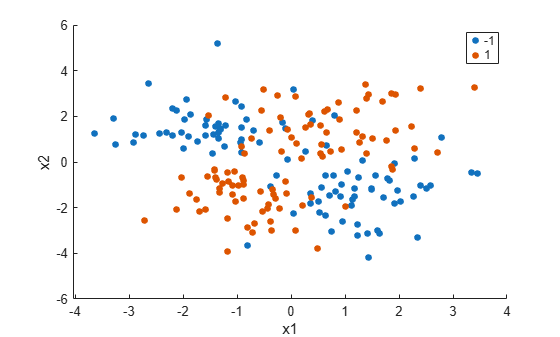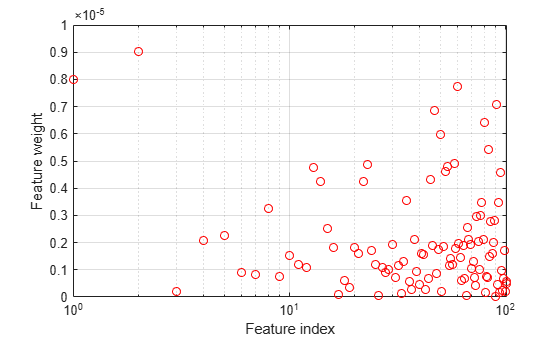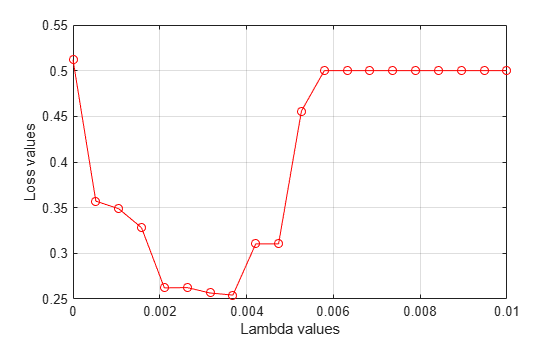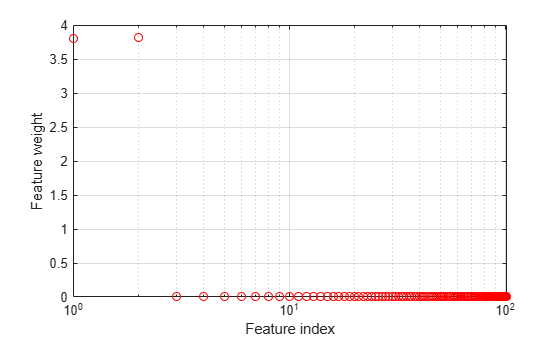Tune Regularization Parameter to Detect Features Using NCA for Classification

This example shows how to tune the regularization parameter in fscnca using cross-validation. Tuning the regularization parameter helps to correctly detect the relevant features in the data.

This dataset is simulated using the scheme described in . This is a two-class classification problem in two dimensions. Data from the first class are drawn from two bivariate normal distributions $N\left({\mu }_{1},\Sigma \right)$ or $N\left({\mu }_{2},\Sigma \right)$ with equal probability, where ${\mu }_{1}=\left[-0.75,-1.5\right]$, ${\mu }_{2}=\left[0.75,1.5\right]$ and $\Sigma ={I}_{2}$. Similarly, data from the second class are drawn from two bivariate normal distributions $N\left({\mu }_{3},\Sigma \right)$ or $N\left({\mu }_{4},\Sigma \right)$ with equal probability, where ${\mu }_{3}=\left[1.5,-1.5\right]$, ${\mu }_{4}=\left[-1.5,1.5\right]$ and $\Sigma ={I}_{2}$. The normal distribution parameters used to create this data set results in tighter clusters in data than the data used in .

Create a scatter plot of the data grouped by the class.

figure
gscatter(X(:,1),X(:,2),y)
xlabel('x1')
ylabel('x2')Add 100 irrelevant features to $X$. First generate data from a Normal distribution with a mean of 0 and a variance of 20.

n = size(X,1);
rng('default')

Normalize the data so that all points are between 0 and 1.

Fit an nca model to the data using the default Lambda (regularization parameter, $\lambda$) value. Use the LBFGS solver and display the convergence information.

ncaMdl = fscnca(X,y,'FitMethod','exact','Verbose',1, ...
'Solver','lbfgs');
o Solver = LBFGS, HessianHistorySize = 15, LineSearchMethod = weakwolfe

|====================================================================================================|
|   ITER   |   FUN VALUE   |  NORM GRAD  |  NORM STEP  |  CURV  |    GAMMA    |    ALPHA    | ACCEPT |
|====================================================================================================|
|        0 |  9.519258e-03 |   1.494e-02 |   0.000e+00 |        |   4.015e+01 |   0.000e+00 |   YES  |
|        1 | -3.093574e-01 |   7.186e-03 |   4.018e+00 |    OK  |   8.956e+01 |   1.000e+00 |   YES  |
|        2 | -4.809455e-01 |   4.444e-03 |   7.123e+00 |    OK  |   9.943e+01 |   1.000e+00 |   YES  |
|        3 | -4.938877e-01 |   3.544e-03 |   1.464e+00 |    OK  |   9.366e+01 |   1.000e+00 |   YES  |
|        4 | -4.964759e-01 |   2.901e-03 |   6.084e-01 |    OK  |   1.554e+02 |   1.000e+00 |   YES  |
|        5 | -4.972077e-01 |   1.323e-03 |   6.129e-01 |    OK  |   1.195e+02 |   5.000e-01 |   YES  |
|        6 | -4.974743e-01 |   1.569e-04 |   2.155e-01 |    OK  |   1.003e+02 |   1.000e+00 |   YES  |
|        7 | -4.974868e-01 |   3.844e-05 |   4.161e-02 |    OK  |   9.835e+01 |   1.000e+00 |   YES  |
|        8 | -4.974874e-01 |   1.417e-05 |   1.073e-02 |    OK  |   1.043e+02 |   1.000e+00 |   YES  |
|        9 | -4.974874e-01 |   4.893e-06 |   1.781e-03 |    OK  |   1.530e+02 |   1.000e+00 |   YES  |
|       10 | -4.974874e-01 |   9.404e-08 |   8.947e-04 |    OK  |   1.670e+02 |   1.000e+00 |   YES  |

Infinity norm of the final gradient = 9.404e-08
Two norm of the final step     = 8.947e-04, TolX   = 1.000e-06
Relative infinity norm of the final gradient = 9.404e-08, TolFun = 1.000e-06
EXIT: Local minimum found.

Plot the feature weights. The weights of the irrelevant features should be very close to zero.

figure
semilogx(ncaMdl.FeatureWeights,'ro')
xlabel('Feature index')
ylabel('Feature weight')
grid onAll weights are very close to zero. This indicates that the value of $\lambda$ used in training the model is too large. When $\lambda \to \infty$, all features weights approach to zero. Hence, it is important to tune the regularization parameter in most cases to detect the relevant features.

Use five-fold cross-validation to tune $\lambda$ for feature selection using fscnca. Tuning $\lambda$ means finding the $\lambda$ value that will produce the minimum classification loss. Here are the steps for tuning $\lambda$ using cross-validation:

1. First partition the data into five folds. For each fold, cvpartition assigns 4/5th of the data as a training set, and 1/5th of the data as a test set.

cvp           = cvpartition(y,'kfold',5);
numtestsets   = cvp.NumTestSets;
lambdavalues  = linspace(0,2,20)/length(y);
lossvalues    = zeros(length(lambdavalues),numtestsets);

2. Train the neighborhood component analysis (nca) model for each $\lambda$ value using the training set in each fold.

3. Compute the classification loss for the corresponding test set in the fold using the nca model. Record the loss value.

4. Repeat this for all folds and all $\lambda$ values.

for i = 1:length(lambdavalues)
for k = 1:numtestsets

% Extract the training set from the partition object
Xtrain = X(cvp.training(k),:);
ytrain = y(cvp.training(k),:);

% Extract the test set from the partition object
Xtest = X(cvp.test(k),:);
ytest = y(cvp.test(k),:);

% Train an nca model for classification using the training set
ncaMdl = fscnca(Xtrain,ytrain,'FitMethod','exact', ...
'Solver','lbfgs','Lambda',lambdavalues(i));

% Compute the classification loss for the test set using the nca
% model
lossvalues(i,k) = loss(ncaMdl,Xtest,ytest, ...

end
end

Plot the average loss values of the folds versus the $\lambda$ values.

figure
plot(lambdavalues,mean(lossvalues,2),'ro-')
xlabel('Lambda values')
ylabel('Loss values')
grid onFind the $\lambda$ value that corresponds to the minimum average loss.

[~,idx] = min(mean(lossvalues,2)); % Find the index
bestlambda = lambdavalues(idx) % Find the best lambda value
bestlambda = 0.0037

Fit the nca model to all of the data using the best $\lambda$ value. Use the LBFGS solver and display the convergence information.

ncaMdl = fscnca(X,y,'FitMethod','exact','Verbose',1, ...
'Solver','lbfgs','Lambda',bestlambda);
o Solver = LBFGS, HessianHistorySize = 15, LineSearchMethod = weakwolfe

|====================================================================================================|
|   ITER   |   FUN VALUE   |  NORM GRAD  |  NORM STEP  |  CURV  |    GAMMA    |    ALPHA    | ACCEPT |
|====================================================================================================|
|        0 | -1.246913e-01 |   1.231e-02 |   0.000e+00 |        |   4.873e+01 |   0.000e+00 |   YES  |
|        1 | -3.411330e-01 |   5.717e-03 |   3.618e+00 |    OK  |   1.068e+02 |   1.000e+00 |   YES  |
|        2 | -5.226111e-01 |   3.763e-02 |   8.252e+00 |    OK  |   7.825e+01 |   1.000e+00 |   YES  |
|        3 | -5.817731e-01 |   8.496e-03 |   2.340e+00 |    OK  |   5.591e+01 |   5.000e-01 |   YES  |
|        4 | -6.132632e-01 |   6.863e-03 |   2.526e+00 |    OK  |   8.228e+01 |   1.000e+00 |   YES  |
|        5 | -6.135264e-01 |   9.373e-03 |   7.341e-01 |    OK  |   3.244e+01 |   1.000e+00 |   YES  |
|        6 | -6.147894e-01 |   1.182e-03 |   2.933e-01 |    OK  |   2.447e+01 |   1.000e+00 |   YES  |
|        7 | -6.148714e-01 |   6.392e-04 |   6.688e-02 |    OK  |   3.195e+01 |   1.000e+00 |   YES  |
|        8 | -6.149524e-01 |   6.521e-04 |   9.934e-02 |    OK  |   1.236e+02 |   1.000e+00 |   YES  |
|        9 | -6.149972e-01 |   1.154e-04 |   1.191e-01 |    OK  |   1.171e+02 |   1.000e+00 |   YES  |
|       10 | -6.149990e-01 |   2.922e-05 |   1.983e-02 |    OK  |   7.365e+01 |   1.000e+00 |   YES  |
|       11 | -6.149993e-01 |   1.556e-05 |   8.354e-03 |    OK  |   1.288e+02 |   1.000e+00 |   YES  |
|       12 | -6.149994e-01 |   1.147e-05 |   7.256e-03 |    OK  |   2.332e+02 |   1.000e+00 |   YES  |
|       13 | -6.149995e-01 |   1.040e-05 |   6.781e-03 |    OK  |   2.287e+02 |   1.000e+00 |   YES  |
|       14 | -6.149996e-01 |   9.015e-06 |   6.265e-03 |    OK  |   9.974e+01 |   1.000e+00 |   YES  |
|       15 | -6.149996e-01 |   7.763e-06 |   5.206e-03 |    OK  |   2.919e+02 |   1.000e+00 |   YES  |
|       16 | -6.149997e-01 |   8.374e-06 |   1.679e-02 |    OK  |   6.878e+02 |   1.000e+00 |   YES  |
|       17 | -6.149997e-01 |   9.387e-06 |   9.542e-03 |    OK  |   1.284e+02 |   5.000e-01 |   YES  |
|       18 | -6.149997e-01 |   3.250e-06 |   5.114e-03 |    OK  |   1.225e+02 |   1.000e+00 |   YES  |
|       19 | -6.149997e-01 |   1.574e-06 |   1.275e-03 |    OK  |   1.808e+02 |   1.000e+00 |   YES  |

|====================================================================================================|
|   ITER   |   FUN VALUE   |  NORM GRAD  |  NORM STEP  |  CURV  |    GAMMA    |    ALPHA    | ACCEPT |
|====================================================================================================|
|       20 | -6.149997e-01 |   5.764e-07 |   6.765e-04 |    OK  |   2.905e+02 |   1.000e+00 |   YES  |

Infinity norm of the final gradient = 5.764e-07
Two norm of the final step     = 6.765e-04, TolX   = 1.000e-06
Relative infinity norm of the final gradient = 5.764e-07, TolFun = 1.000e-06
EXIT: Local minimum found.

Plot the feature weights.

figure
semilogx(ncaMdl.FeatureWeights,'ro')
xlabel('Feature index')
ylabel('Feature weight')
grid onfscnca correctly figures out that the first two features are relevant and the rest are not. Note that the first two features are not individually informative but when taken together result in an accurate classification model.

References

1. Yang, W., K. Wang, W. Zuo. "Neighborhood Component Feature Selection for High-Dimensional Data." Journal of Computers. Vol. 7, Number 1, January, 2012.